Thermodynamic Magic Square

# Thermodynamic Magic Square | Physical Chemistry

 1 Crore+ students have signed up on EduRev. Have you?

Maxwell Thermodynamic Equations

In thermodynamics, the Maxwell equations are a set of equations derived by application of Euler's reciprocity relation to the thermodynamic characteristic functions.

The Maxwell relations, first derived by James Clerk Maxwell, are the following expressions:

• dH = TdS + VdP
• dG = –SdT + VdP
• dA = –PdV – SdT
• dV = TdS – PdV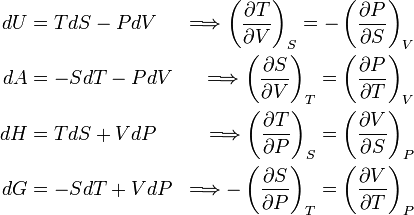Thermodynamic Magic Square

The magic square plays a very important role in thermodynamic. By using magic square we find many important relationships between thermodynamic function or quantity.

These relations are known as Maxwell thermodynamic equations. S, P, V, T are at the corner of the square & known as thermodynamic coordinates.
We can find the Maxwell thermodynamic equation by using magic square.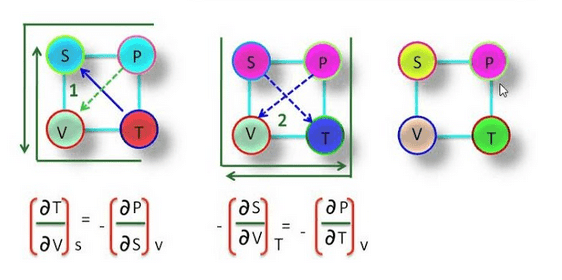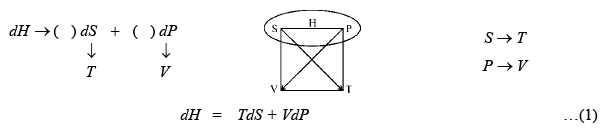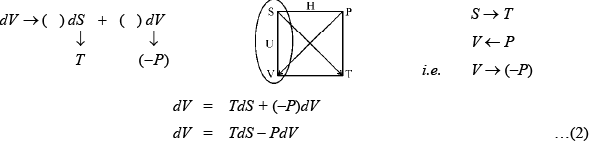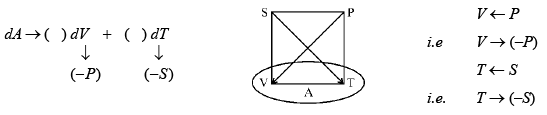dA = (–P)dV + (–S)dT
dA = –PdV – SdT …(3)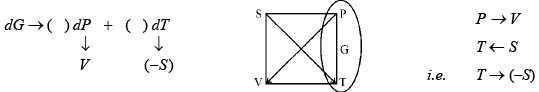dG = VdP + (–S)dT
dG = VdP – SdT …(4) i.e.,

we can find the Maxwell thermodynamic equation by using magic square.

Maxwell Relationship between Thermodynamic Coordinates
i.e., S, P, V, T.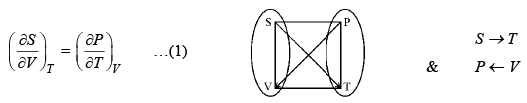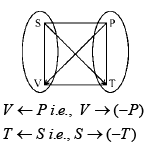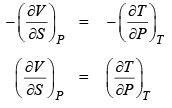..........(2)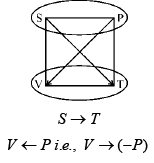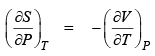..............(3)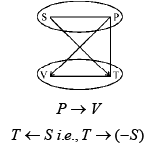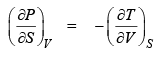................(4)

We can find the relation between thermodynamic coordinates by using Euler’s theorem.
We know that if z s the function of x & y then z = f(x, y)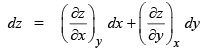if z is a state function then it follows Euler’s theorem
i.e.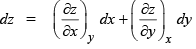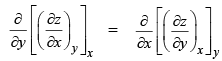Four Maxwell thermodynamic equation are given below: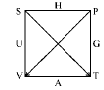dH = TdS + VdP
dG = VdP – SdT
dA = –PdV – SdT
dV = TdS – PdV

We know that H, G, A & V are state function i.e., it follow the Euler’s theorem.

dH = TdS + VdP

i.e.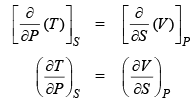dG = VdP – SdT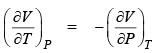dA = –PdV – SdTi.e.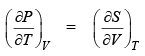dV = TdS – PdV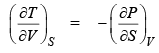Expansivity and Compressibility

The gas expands on heating through the expansion of gases is much more than that of liquids.  Similar gas compressed on increasing the pressure.

“The variation of volume V with temperature T, keeping pressure P constant is called the coefficient of thermal expansion or expansivity. It is denoted by α.  Thus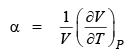Similarly “the variation of V with P, keeping T constant, is called the coefficient of isothermal compressibility or compressibility. It is denoted by β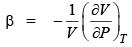Another coefficient, isochoric thermal expansion coefficient, when the variation of P with T, keeping V constant is represented by γ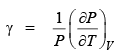Q.1.  Find the value of α, β, γ for an ideal gas?
Sol. Ideal gas equation is
PV = nRT         (for n mole)

PV = RT           (for 1 mole)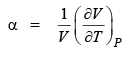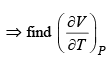for ideal gas

PV = nRT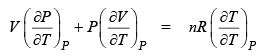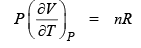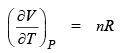…(1)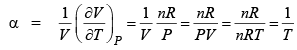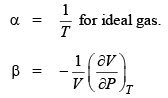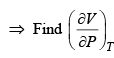for ideal gas

PV = nRT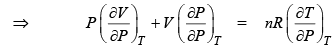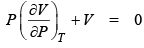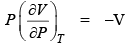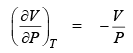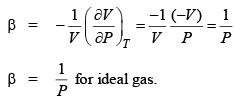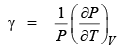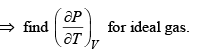PV = nRT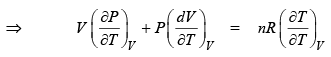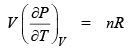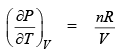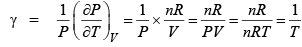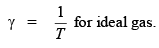The document Thermodynamic Magic Square | Physical Chemistry is a part of the Chemistry Course Physical Chemistry.
All you need of Chemistry at this link: Chemistry

## Physical Chemistry

85 videos|131 docs|67 tests

## Physical Chemistry

85 videos|131 docs|67 tests

Track your progress, build streaks, highlight & save important lessons and more!(Scan QR code)

,

,

,

,

,

,

,

,

,

,

,

,

,

,

,

,

,

,

,

,

,

;